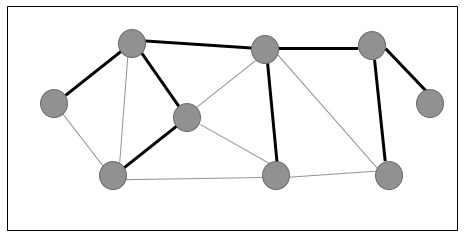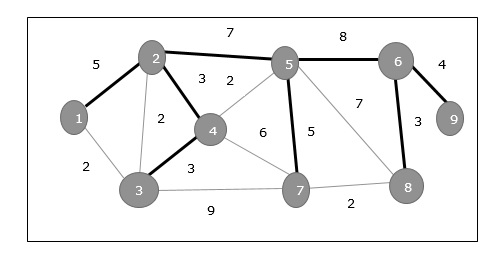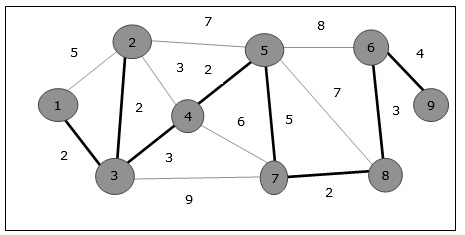# Design and Analysis - Spanning Tree

A spanning tree is a subset of an undirected Graph that has all the vertices connected by minimum number of edges.

If all the vertices are connected in a graph, then there exists at least one spanning tree. In a graph, there may exist more than one spanning tree.

### Properties

• A spanning tree does not have any cycle.

• Any vertex can be reached from any other vertex.

### Example

In the following graph, the highlighted edges form a spanning tree.## Minimum Spanning Tree

A Minimum Spanning Tree (MST) is a subset of edges of a connected weighted undirected graph that connects all the vertices together with the minimum possible total edge weight. To derive an MST, Prim’s algorithm or Kruskal’s algorithm can be used. Hence, we will discuss Prim’s algorithm in this chapter.

As we have discussed, one graph may have more than one spanning tree. If there are n number of vertices, the spanning tree should have 𝒏−𝟏 number of edges. In this context, if each edge of the graph is associated with a weight and there exists more than one spanning tree, we need to find the minimum spanning tree of the graph.

Moreover, if there exist any duplicate weighted edges, the graph may have multiple minimum spanning tree.In the above graph, we have shown a spanning tree though it’s not the minimum spanning tree. The cost of this spanning tree is (5 + 7 + 3 + 3 + 5 + 8 + 3 + 4) = 38.

We will use Prim’s algorithm to find the minimum spanning tree.

## Prim’s Algorithm

Prim’s algorithm is a greedy approach to find the minimum spanning tree. In this algorithm, to form a MST we can start from an arbitrary vertex.

Algorithm: MST-Prim’s (G, w, r)
for each u є G.V
u.key = ∞
u.Π = NIL
r.key = 0
Q = G.V
while Q ≠ф
u = Extract-Min (Q)
if each v є Q and w(u, v) <v.key
v.Π = u
v.key = w(u, v)


The function Extract-Min returns the vertex with minimum edge cost. This function works on min-heap.

### Example

Using Prim’s algorithm, we can start from any vertex, let us start from vertex 1.

Vertex 3 is connected to vertex 1 with minimum edge cost, hence edge (1, 2) is added to the spanning tree.

Next, edge (2, 3) is considered as this is the minimum among edges {(1, 2), (2, 3), (3, 4), (3, 7)}.

In the next step, we get edge (3, 4) and (2, 4) with minimum cost. Edge (3, 4) is selected at random.

In a similar way, edges (4, 5), (5, 7), (7, 8), (6, 8) and (6, 9) are selected. As all the vertices are visited, now the algorithm stops.

The cost of the spanning tree is (2 + 2 + 3 + 2 + 5 + 2 + 3 + 4) = 23 . There is no more spanning tree in this graph with cost less than 23.